Related Articles

# Filter Pandas Dataframe with multiple conditions

• Last Updated : 16 Mar, 2021

In this article, let’s discuss how to filter pandas dataframe with multiple conditions. There are possibilities of filtering data from Pandas dataframe with multiple conditions during the entire software development. The reason is dataframe may be having multiple columns and multiple rows. Selective display of columns with limited rows is always the expected view of users. To fulfill the user’s expectations and also help in machine deep learning scenarios, filtering of Pandas dataframe with multiple conditions is much necessary.

Let us see the different ways to do the same.

Creating a sample dataframe to proceed further

## Python3

 `# import module``import` `pandas as pd`` ` `# assign data``dataFrame ``=` `pd.DataFrame({``'Name'``: [``' RACHEL  '``, ``' MONICA  '``, ``' PHOEBE  '``,``                                   ``'  ROSS    '``, ``'CHANDLER'``, ``' JOEY    '``],``                           ` `                          ``'Age'``: [``30``, ``35``, ``37``, ``33``, ``34``, ``30``],``                           ` `                          ``'Salary'``: [``100000``, ``93000``, ``88000``, ``120000``, ``94000``, ``95000``],``                           ` `                          ``'JOB'``: [``'DESIGNER'``, ``'CHEF'``, ``'MASUS'``, ``'PALENTOLOGY'``,``                                  ``'IT'``, ``'ARTIST'``]})`` ` `# display dataframe``display(dataFrame)`

Output: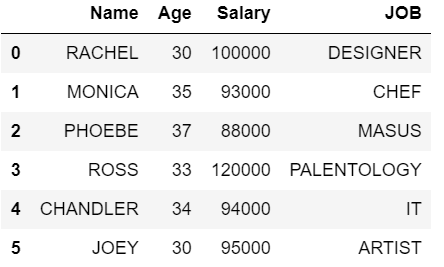Method 1: Using loc

Here we will get all rows having Salary greater or equal to 100000 and Age < 40 and their JOB starts with ‘D’ from the dataframe. Print the details with Name and their JOB. For the above requirement, we can achieve this by using loc. It is used to access single or more rows and columns by label(s) or by a boolean array. loc works with column labels and indexes.

## Python3

 `# import module``import` `pandas as pd`` ` `# assign data``dataFrame ``=` `pd.DataFrame({``'Name'``: [``' RACHEL  '``, ``' MONICA  '``, ``' PHOEBE  '``,``                                   ``'  ROSS    '``, ``'CHANDLER'``, ``' JOEY    '``],``                           ` `                          ``'Age'``: [``30``, ``35``, ``37``, ``33``, ``34``, ``30``],``                           ` `                          ``'Salary'``: [``100000``, ``93000``, ``88000``, ``120000``, ``94000``, ``95000``],``                           ` `                          ``'JOB'``: [``'DESIGNER'``, ``'CHEF'``, ``'MASUS'``, ``'PALENTOLOGY'``,``                                  ``'IT'``, ``'ARTIST'``]})``# filter dataframe``display(dataFrame.loc[(dataFrame[``'Salary'``]>``=``100000``) & (dataFrame[``'Age'``]< ``40``) & (dataFrame[``'JOB'``].``str``.startswith(``'D'``)),``                    ``[``'Name'``,``'JOB'``]])`

Output: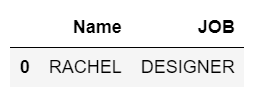Output resolves for the given conditions and finally, we are going to show only 2 columns namely Name and JOB.

Method 2: Using NumPy

Here will get all rows having Salary greater or equal to 100000 and Age < 40 and their JOB starts with ‘D’ from the data frame. We need to use NumPy.

## Python3

 `# import module``import` `pandas as pd``import` `numpy as np`` ` `# assign data``dataFrame ``=` `pd.DataFrame({``'Name'``: [``' RACHEL  '``, ``' MONICA  '``, ``' PHOEBE  '``,``                                   ``'  ROSS    '``, ``'CHANDLER'``, ``' JOEY    '``],``                           ` `                          ``'Age'``: [``30``, ``35``, ``37``, ``33``, ``34``, ``30``],``                           ` `                          ``'Salary'``: [``100000``, ``93000``, ``88000``, ``120000``, ``94000``, ``95000``],``                           ` `                          ``'JOB'``: [``'DESIGNER'``, ``'CHEF'``, ``'MASUS'``, ``'PALENTOLOGY'``,``                                  ``'IT'``, ``'ARTIST'``]})`` ` `# filter dataframe                                   ``filtered_values ``=` `np.where((dataFrame[``'Salary'``]>``=``100000``) & (dataFrame[``'Age'``]< ``40``) & (dataFrame[``'JOB'``].``str``.startswith(``'D'``)))``print``(filtered_values)``display(dataFrame.loc[filtered_values])`

Output: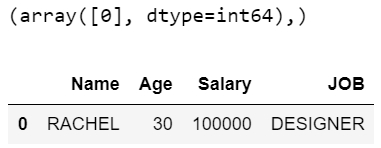In the above example, print(filtered_values) will give the output as (array(, dtype=int64),)  which indicates the first row with index value 0 will be the output. After that output will have 1 row with all the columns and it is retrieved as per the given conditions.

Method 3: Using Query (eval and query works only with columns)

In this approach, we get all rows having Salary lesser or equal to 100000 and Age < 40, and their JOB starts with ‘C’ from the dataframe. Its just query the columns of a DataFrame with a single or more Boolean expressions and if multiple, it is having & condition in the middle.

## Python3

 `# import module``import` `pandas as pd`` ` `# assign data``dataFrame ``=` `pd.DataFrame({``'Name'``: [``' RACHEL  '``, ``' MONICA  '``, ``' PHOEBE  '``,``                                   ``'  ROSS    '``, ``'CHANDLER'``, ``' JOEY    '``],``                           ` `                          ``'Age'``: [``30``, ``35``, ``37``, ``33``, ``34``, ``30``],``                           ` `                          ``'Salary'``: [``100000``, ``93000``, ``88000``, ``120000``, ``94000``, ``95000``],``                           ` `                          ``'JOB'``: [``'DESIGNER'``, ``'CHEF'``, ``'MASUS'``, ``'PALENTOLOGY'``,``                                  ``'IT'``, ``'ARTIST'``]})`` ` `# filter dataframe ``display(dataFrame.query(``'Salary  <= 100000 & Age < 40 & JOB.str.startswith("C").values'``))`

Output: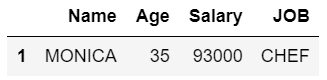Method 4: pandas Boolean indexing multiple conditions standard way (“Boolean indexing” works with values in a column only)

In this approach, we get all rows having Salary lesser or equal to 100000 and Age < 40 and their JOB starts with ‘P’ from the dataframe. In order to select the subset of data using the values in the dataframe and applying Boolean conditions, we need to follow these ways

## Python3

 `# import module``import` `pandas as pd`` ` `# assign data``dataFrame ``=` `pd.DataFrame({``'Name'``: [``' RACHEL  '``, ``' MONICA  '``, ``' PHOEBE  '``,``                                   ``'  ROSS    '``, ``'CHANDLER'``, ``' JOEY    '``],``                           ` `                          ``'Age'``: [``30``, ``35``, ``37``, ``33``, ``34``, ``30``],``                           ` `                          ``'Salary'``: [``100000``, ``93000``, ``88000``, ``120000``, ``94000``, ``95000``],``                           ` `                          ``'JOB'``: [``'DESIGNER'``, ``'CHEF'``, ``'MASUS'``, ``'PALENTOLOGY'``,``                                  ``'IT'``, ``'ARTIST'``]})`` ` `# filter dataframe ``display(dataFrame[(dataFrame[``'Salary'``]>``=``100000``) & (dataFrame[``'Age'``]<``40``) & dataFrame[``'JOB'``].``str``.startswith(``'P'``)][[``'Name'``,``'Age'``,``'Salary'``]])`

Output: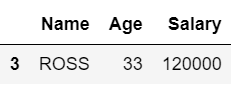We are mentioning a list of columns that need to be retrieved along with the Boolean conditions and since many conditions, it is having ‘&’.

Method 5: Eval multiple conditions  (“eval” and “query” works only with columns )

Here, we get all rows having Salary lesser or equal to 100000 and Age < 40 and their JOB starts with ‘A’ from the dataframe.

## Python3

 `# import module``import` `pandas as pd`` ` `# assign data``dataFrame ``=` `pd.DataFrame({``'Name'``: [``' RACHEL  '``, ``' MONICA  '``, ``' PHOEBE  '``,``                                   ``'  ROSS    '``, ``'CHANDLER'``, ``' JOEY    '``],``                           ` `                          ``'Age'``: [``30``, ``35``, ``37``, ``33``, ``34``, ``30``],``                           ` `                          ``'Salary'``: [``100000``, ``93000``, ``88000``, ``120000``, ``94000``, ``95000``],``                           ` `                          ``'JOB'``: [``'DESIGNER'``, ``'CHEF'``, ``'MASUS'``, ``'PALENTOLOGY'``,``                                  ``'IT'``, ``'ARTIST'``]})`` ` `# filter dataframe ``display(dataFrame[dataFrame.``eval``(``"Salary <=100000 & (Age <40) & JOB.str.startswith('A').values"``)])`

Output: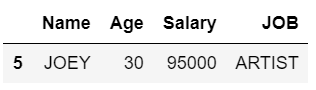Dataframes are a very essential concept in Python and filtration of data is required can be performed based on various conditions. They can be achieved in any one of the above ways. Ponts to be noted:

• loc works with column labels and indexes.
• eval and query works only with columns.
• Boolean indexing works with values in a column only.

Attention geek! Strengthen your foundations with the Python Programming Foundation Course and learn the basics.

To begin with, your interview preparations Enhance your Data Structures concepts with the Python DS Course. And to begin with your Machine Learning Journey, join the Machine Learning – Basic Level Course

My Personal Notes arrow_drop_up